##### Statistics For Dummies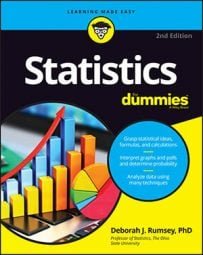You can find probabilities for a sample proportion by using the normal approximation as long as certain conditions are met. For example, say that a statistical study claims that 0.38 or 38% of all the students taking the ACT test would like math help. Suppose you take a random sample of 100 students. What is the probability that more than 45 of them say they need math help? In terms of proportions, this is equivalent to the probability that more than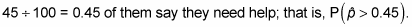To answer this question, you first check the conditions: First, is np (sample size * population proportion) at least 10? Yes, because 100 ∗ 0.38 = 38. Next, is n(1 – p) at least 10? Again yes, because 100 ∗ (1 – 0.38) = 62 checks out. So you can go ahead and use the normal approximation.

You make the conversion of the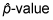to a z-value using the following general equation: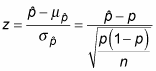When you plug in the numbers for this example, you get: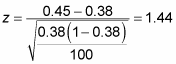It is very important that you pay attention to which value reflects the population proportion p and which value was calculated as the sample proportion, p-hat. Flip-flopping them in the formula for z would result in a vastly different answer.

And then you find P(Z > 1.44) using the following table.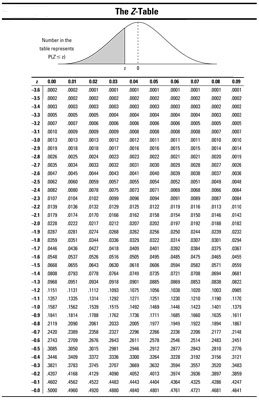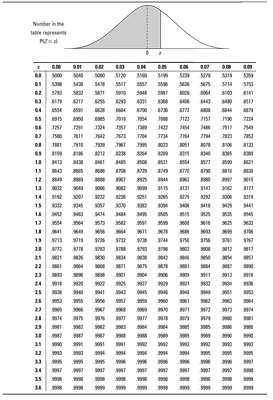From the table, you determine that P(Z > 1.44) = 1 – 0.9251 = 0.0749. So if it’s true that 38 percent of all students taking the exam want math help, then in a random sample of 100 students the probability of finding more than 45 needing math help is approximately 0.0749 (by the Central Limit Theorem).

You can use sample proportions to check out a claim about a population proportion. (This procedure is a hypothesis test for a population proportion.) In the ACT example, the probability that more than 45% of the students in a sample of 100 need math help (when you assumed 38% of the population needed math help) was found to be 0.0749. Because this probability is higher than 0.05 (the typical cutoff for blowing the whistle on a claim about a population value), you can’t dispute their claim that the percentage in the population needing math help is only 38%. This sample result is just not a rare enough event.